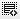liquidation preference0
Assume an Investor who invested s100 million hold 15per cent in your co and has a 2x liquidation preference. if you sell your co for s2billion, how much will the investor get?0

profit from the co. sold
=2x multiply by 0.15 x S2billion = 600million
600million - 100million( money invested)
=500million.
2x (0.15) x 100million = 30million
S500million - S30million = S470million
Investor will get S470million0

2x Liquidation Preference=2*100= 200m
15% of (2000-200) = 270m
Hence
200m+270= 470m

Click on thisicon to add code snippet.